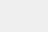# 深度强化学习专栏（三）1. 引言

• 专栏知识结构

• 从AlphaGo看深度强化学习

2. 强化学习基础知识

• 强化学习问题

• 马尔科夫决策过程

• 最优价值函数和贝尔曼方程

• 价值迭代

• 策略迭代

4. 无模型的强化学习方法

• 蒙特卡洛方法

• 时序差分学习

• 值函数近似

• 策略搜索

5. 实战强化学习算法

• Q-learning 算法

• Monte Carlo Policy Gradient 算法

• Actor Critic 算法

6. 深度强化学习算法

• Deep Q-Networks(DQN)

7. 专栏小结

## 4.1 蒙特卡洛方法

1.策略估计和策略改进``Initialization：Initial a polic πFor s in S:    For a in A:        Initial Q(s,a) and Return(s,a)        c(s,a)=0For i in range(k):Generate an episode using πFor each state s appearing in the episode:G ← the return that follows the first occurrence of sQ(s_t,a_t)=(c(s_t,a_t)*Q(s_t,a_t)+G)/(c(s_t,a_t)+1)c(s_t,a_t)++end forupdate policy π(s)=argmaxQ(s,a)end for``

2.环境探索

ε贪心搜索方法：首先初始一个概率ε，在进行策略估计的时候，以ε的概率随机选择一个动作，以1-ε的概率去根据当前的策略选择动作。考虑到刚开始进行策略估计的时候，应该更注重对环境的探索，而随着策略慢慢地改进，则应该更注重策略的利用。所以，初始的ε值较大，随着策略的不断改进，慢慢减小ε的值。

3.确定性的和非确定性的环境状态
“Frozen Lake”游戏有“无风”和“有风”两种游戏模式。“无风”模式下，游戏环境是确定的，即agent执行一个动作（例如右移或下移）后，到达的下一个状态是确定的，并且得到的奖励也是确定的，而在“有风”模式下，环境状态则不确定了。图6-10中的蒙特卡洛算法考虑的是非确定性的环境状态，所以采用取平均值的方式，希望能够近似替代真实的状态价值。如果是确定性的环境状态的话，就可以省去取平均值的操作，直接赋值为：Q(s_t,a_t)=G。

4.在策略（on-policy）和离策略（off-policy）

## 4.2 时序差分学习

1. 蒙特卡洛更新和时序差分更新2. Sarsa算法
Sarsa算法是时序差分学习的在策略版本，Sarsa算法的伪代码如下：
``For s in S:For a in A:    Initialize Q(s,a)For i in range(k):Initialize SChoose a using policy derived from Q (e.g.ε-greedy)Repeat (for each step of episode):Take action a,observe r and sChoose a^' using policy derived from Q (e.g.ε-greedy)Q(s, a) ← Q(s, a) + α[R + γQ(s^', a^') − Q(s, a)]s ←s^'; a ←a^'until s is terminalEnd for``
Sarsa算法
3. Q-Learning算法
Q-Learning算法是时序差分学习方法的离策略版本，Q-Learning算法的伪代码如下：
``For s in S:For a in A:    Initialize Q(s,a)For i in range(k):Initialize SRepeat (for each step of episode):Choose a using policy derived from Q (e.g.ε-greedy)Take action a,observe r and sQ(s, a) ← Q(s, a) + α[R + γmax(Q(s^', a^')) − Q(s, a)]s ←s^'until s is terminalEnd for``
Q-Learning算法## 4.3 值函数近似## 4.4 策略搜索

1. 基于值函数（value-based）和基于策略函数（policy-based）``Input: a differentiable policy parameterization π_θ (a|s)Initialize policy parameter θRepeat:Generate an episode τ: S_0,A_0,R_1,…, S_(T-1), A_(T-1), R_T, following π_θ (a|s)For each step of the episode t = 0,…,T-1:G ← return from step t                  θ← θ+ 〖αγ〗^t G(τ_(t:T) ) ∂/∂θ logπ_θ (a_t |s_t)    EndUntil θ is convergedOutput: π_θ``
4. Actor Critic算法

``Input: a differentiable policy parameterization π_θ (a|s)Input: a differentiable state-value parameterization V_∅ (s)Repeat:Initialize state s         ℷ=1  Repeat:      On state s, choose an action a=π_θ (a|s)      Execute a, get reward r and new state s^'      δ←r+γV_∅ (s^' )-V_∅ (s)      ∅←∅+βλδ ∂/(∂∅) V_∅ (s) #更新值函数的参数      θ←θ+αλδ ∂/∂θ logπ_θ (a|s) #更新策略函数的参数      λ←γλ      s←s^'  until s is terminaluntil θ is convergedOutput: π_θ``
actor-critic算法

●
● 深度强化学习（DRL）专栏开篇
●点击下方 |  | 了解更多